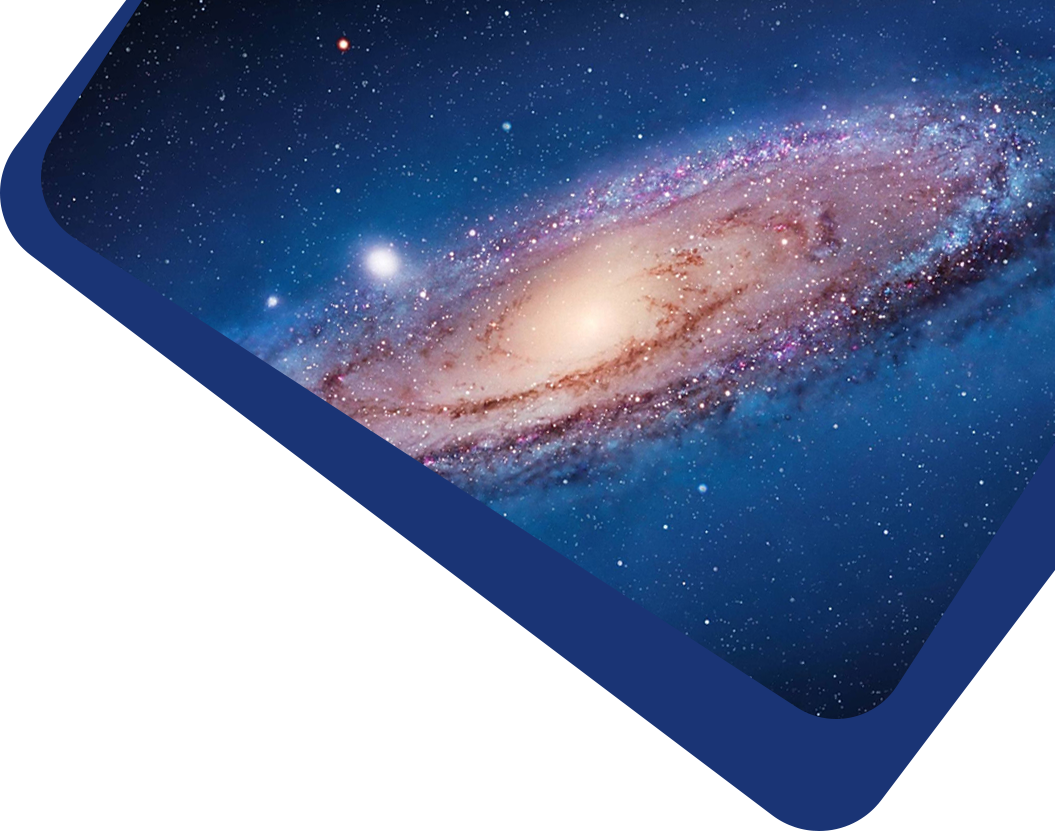Vol 23, No 2

## Simulation-driven Wind Load Analysis and Prediction for Large Steerable Radio Telescopes

#### Shuai Li, Bao-Qing Han, Shao-Yi Liu, Wen-Juan Wang, Ben-Ben Wang, Xin-Yu Zhang, Long-Yang Wang, Qian Xu, Na Wang, Wu-Lin Zhao et al

Abstract

Large steerable radio telescopes can rotate in azimuth and elevation, with various upwind postures and complex wind load characteristics. In order to obtain the wind load distribution on the reflector and the wind force coefficients in different upwind postures, this work took the reflector of the QiTai Telescope in Xinjiang as the object. The wind pressure distribution, drag coefficient, side force coefficient, lift coefficient, and total force coefficient of 361 groups of reflectors with different upwind postures were calculated and analyzed by a numerical simulation method. The results show that the force on the reflector when the concave surface faces the wind is significantly greater than when the convex surface faces the wind. The surrogate models for calculating wind force coefficients were established based on the polynomial response surface model (PRSM) and regularized minimum-energy tensor-product spline (RMTS). The adjusted R-squared of RMTS is 0.98, and the root mean square error is below 0.1, which proves RMTS is significantly better than PRSM. Compared with the numerical simulation values, the absolute errors of the drag coefficient, side force coefficient, lift coefficient, and total force coefficient of the reflector with azimuth and elevation of 45° predicted by RMTS are 0.02, 0.027, 0.032, and 0.046, respectively. The relative errors are 2.42%, 2.76%, 2.23%, and 2.43% , respectively. It is proved that the RMTS surrogate model is reliable and that the predicted data can fast provide sufficient information for the wind-resistant design of the large steerable radio telescopes.

Keywords

methods: numerical – methods: data analysis – telescopes – atmospheric effects

Full Text
Refbacks